## python matplotlib 画图整理_matplotlib 每次调用函数画一张图_angnuan123的博客-程序员秘密

## plot 板块##

import matplotlib.pyplot as plt
import numpy as np

x=np.linspace(1,10,10)
y=x
y1=2*x**2+1
y2=2*x**3+1

# 使用figure()函数重新申请一个figure对象
# 注意，每次调用figure的时候都会重新申请一个figure对象
plt.figure()

plt.plot(x,y)

# 第一个参数表示的是编号，第二个表示的是图表的长宽
plt.figure(num = 3, figsize=(8, 5))

# 当我们需要在画板中绘制两条线的时候，可以使用下面的方法：
l1,= plt.plot(x, y2,label='bbb')
l2,= plt.plot(x, y1,
color='red',   # 线颜色
linewidth=1.0,  # 线宽
linestyle='--',  # 线样式
label='aaa'
)
#首先不知道为啥非要加上上述的','其次如果后面指定了新的label,以新的为主
plt.legend(handles=[l1,l2],
labels=[r'aaa',r'ddd'],
loc='best')#loc：1，2，3，4，从右上角逆时针

# 设置轴线的lable（标签）
plt.xlabel("I am x")
plt.ylabel("I am y")

#设置取值参数范围：
plt.xlim((-1,2))
plt.ylim((1,3))

#设置坐标轴点的位置
new_ticks= np.linspace(-1,2,5)
plt.xticks(new_ticks)
#y 轴对应点加上我们想要的文字：加上$可以倾斜 plt.yticks([-2,-1.8,-1,1.22,5], [r'$really\ bad$',r'$bad$',r'normal','$good$','really good']) #gca = 'get current axis' 主要是为了对接下来的一些东西做设定 ax=plt.gca() #将右边和上面的边框颜色去掉： ax.spines['right'].set_color('none') ax.spines['top'].set_color('none') #设置x轴和y轴的显示位置： ax.xaxis.set_ticks_position('bottom')#(指的是整个图的下面) ax.yaxis.set_ticks_position('right') #定义x,y轴在图像中坐标位置： ax.spines['bottom'].set_position(('data',0))#'bottom'位于y=0 ax.spines['left'].set_position(('data',0.5))#'left'位于=1 #批注，显示交叉点 x0=1 y0=2*x0+1 plt.scatter(x0,y0,s=66,color='r')#s表示点的大小 plt.plot([x0,x0],[y0,0],'k-.',lw=2.5) #设置关键提示信息 plt.annotate(r'$2x+1=%s$'%y0, xy=(x0,y0), xycoords='data', xytext=(+30,-30), textcoords='offset points', fontsize=16, #设置箭头： arrowprops=dict(arrowstyle='->',connectionstyle='arc3,rad=.2') ) #用于显示文字： plt.text(0,3, r'$This\ is\ a\ good\ idea.\ \mu\ \sigma_i$', fontdict={'size':16,'color':'g'}) plt.show()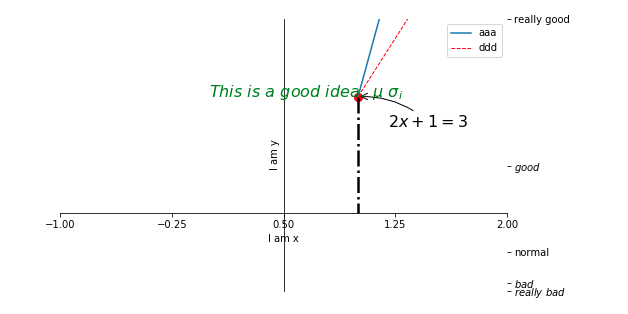## 条状图## import numpy as np import matplotlib.pyplot as plt mu, sigma = 100, 15 x = mu + sigma * np.random.randn(10000) # the histogram of the data n, bins, patches = plt.hist(x, 50, density=1, facecolor='g', alpha=0.75) plt.xlabel('Smarts') plt.ylabel('Probability') plt.title('Histogram of IQ') plt.text(60, .025, r'$\mu=100,\ \sigma=15\$')
plt.axis([40, 160, 0, 0.03])
plt.grid(True)
plt.show()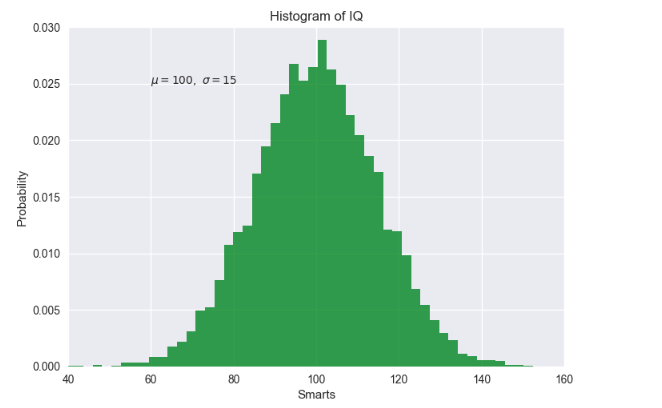import matplotlib.pyplot as plt
import numpy as np

n = 12
X = np.arange(n)
Y1 = (1 - X/float(n)) * np.random.uniform(0.5, 1.0, n)
Y2 = (1 - X/float(n)) * np.random.uniform(0.5, 1.0, n)

plt.figure(figsize=(12, 8))
plt.bar(X, +Y1, facecolor='#9999ff', edgecolor='white')
plt.bar(X, -Y2, facecolor='#ff9999', edgecolor='white')

for x, y in zip(X,Y1):
# ha: horizontal alignment水平方向
# va: vertical alignment垂直方向
plt.text(x, y+0.05, '%.2f' % y, ha='center', va='bottom')

for x, y in zip(X,-Y2):
# ha: horizontal alignment水平方向
# va: vertical alignment垂直方向
plt.text(x, y-0.05, '%.2f' % y, ha='center', va='top')

# 定义范围和标签
plt.xlim(-.5, n)
plt.xticks(())
plt.ylim(-1.25, 1.25)
plt.yticks(())

plt.show()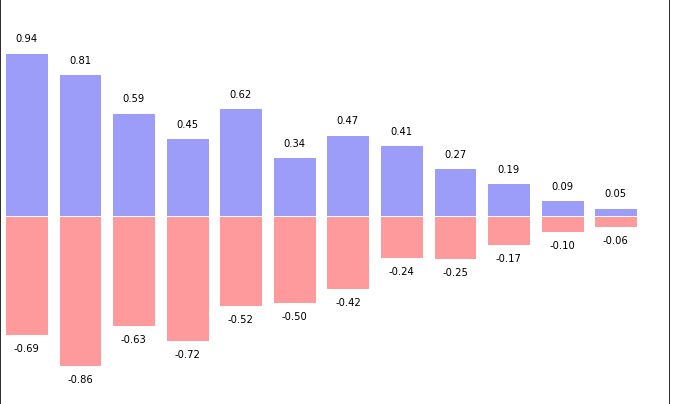##  subplot 板块##

import matplotlib.pyplot as plt
import numpy as np

plt.figure()

# 将整个figure分成两行三列
plt.subplot(2, 3, 1)
# 第一个参数表示X的范围，第二个是y的范围
plt.plot([0, 1], [0, 2])

#表示两行三列中的第一个位置，按照行先排序
plt.subplot(231)
plt.plot([0, 1], [0, 2])

plt.subplot(232)
plt.plot([0, 2], [0, 4])

plt.subplot(234)
plt.plot([0, 1], [0, 3])

plt.subplot(236)
plt.plot([0, 1], [0, 4])

plt.show()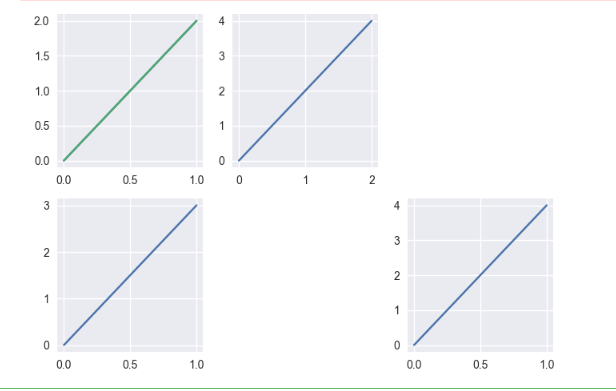###分格显示和主次坐标轴看原微博###

##箱线图板块##

boxes, 是25分位值和75分位值构成的box,
medians, 是中位值的横线, 每个median是一个Line2D对象
whiskers, 是指从box 到error bar之间的竖线.
fliers, 是指error bar线之外的离散点.
caps, 是指error bar横线.
means, 是均值的横线,

import numpy as np
import matplotlib.pyplot as plt
import pandas as pd
np.random.seed(2)  #设置随机种子
df = pd.DataFrame(np.random.rand(5,4),
columns=['A', 'B', 'C', 'D'])#先生成0-1之间的5*4维度数据，再装入4列DataFrame中
df.boxplot() #也可用plot.box()
plt.show()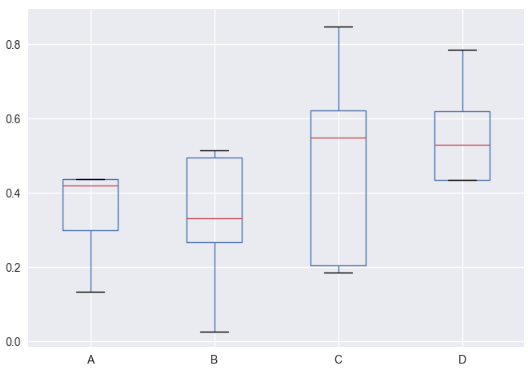f=df.boxplot(sym='r*',patch_artist=True,return_type='dict')
#没有return_type='dict' 会报错：TypeError: 'AxesSubplot' object is not subscriptable

for box in f['boxes']:
# 箱体边框颜色
box.set( color='#7570b3', linewidth=2)
# 箱体内部填充颜色
box.set( facecolor = '#1b9e77' )
for whisker in f['whiskers']:
whisker.set(color='r', linewidth=2)
for cap in f['caps']:
cap.set(color='g', linewidth=3)
for median in f['medians']:
median.set(color='DarkBlue', linewidth=3)
for flier in f['fliers']:
flier.set(marker='o', color='y', alpha=0.5)
plt.show()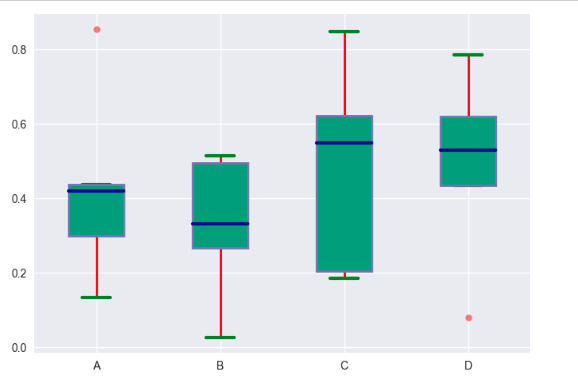#横向：
df.boxplot(sym='r*',vert=False,patch_artist=True,meanline=False,showmeans=True)
plt.show()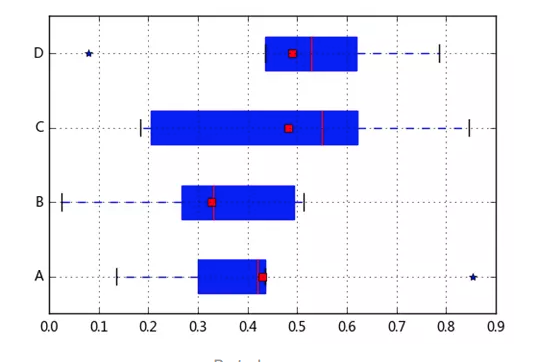vert=False，表示横向还是竖向（True），,
patch_artist=True,（上下四分位框内是否填充，True为填充）
meanline=False,showmeans=True，是否有均值线及其形状，meanline=True时，均值线也像中位数线一样是条红色线段，这样容易与中位数线混淆。

color:

one of {'b', 'g', 'r', 'c', 'm', 'y', 'k', 'w'};

linewidth（lw）:直接写数字

linstyle：'--','k-.'

lineStyles = {'': '_draw_nothing', ' ': '_draw_nothing', '-': '_draw_solid', '--': '_draw_dashed', '-.': '_draw_dash_dot', ':': '_draw_dotted', 'None': '_draw_nothing'}

loc：'best',1，2，3，4

==========关闭图：===========

   clf() # 清图。
cla() # 清坐标轴。
close() # 关窗口

【e.g.】
fig = plt.figure() # 新图 0
plt.savefig() # 保存
plt.close('all') # 关闭图 0

### ubuntu下　利用ｓｈ文件实现在多个终端下快速执行命令_m_zhangJingDong的博客-程序员秘密

ｕｂｕｎｔｕ下，启动一个程序可能需要打开多个终端输入多次命令，反正我觉得这样是太麻烦了，写了个ｓｈ文件，直接运行就号了，需要注意的是，一定要给写好的ｓｈ文件足够的权限：　　　　　　　ｓｕｄｏ chmod -R 777 ｘｘｘ.sh下面给个ｄｅｍｏ：　#!/bin/bashsource ~/catkin_ws1/devel/setup.bash{gnome-terminal -t "XXD_

### CentOS设置SSH Key登录_keneuro的博客-程序员秘密

SSH是我们登录VPS常用的方式，因此SSH账号的安全至关重要。常见的用户名+密码的登录方式很容易受到暴力破解的影响，为了避免影响，我们可以直接禁止用户名+密码登录VPS的方式，改用密钥登录，既保证了安全，又方便快捷。下面就介绍一下配置ssh key登录的方法。一、PuttyGen以及Putty软件的下载这一步看似简单，其实有着至关重要的作用。大家一定要到官方网站进行下载（putt

### VC 开发ocx_c++ ocx_FrankieWang008的博客-程序员秘密

[转]基于MFC的ActiveX控件开发作者：lidan | 出处：博客园 | 2012/3/13 16:10:34 | 阅读22次ActiveX 控件是基于组件对象模型 (COM) 的可重用软件组件，广泛应用于桌面及Web应用中。在VC下ActiveX控件的开发可以分为三种，一种是直接用COM的API来开发，这样做显然非常的麻烦，对程序员要求也非常高，因此一般是不予考虑的；一

### 极其简单的手机安装及部署maven应用_apt安装maven_影alter的博客-程序员秘密

aidlux maven 手机 服务器 手机服务器教程

### Mybatis-plus介绍及使用_小码哥呀的博客-程序员秘密

mybatis-plus官方教程:https://mp.baomidou.com/guide/Mybatis-plus介绍及使用1、Mybatis-plus简介2、Mybatis-plus坐标3、Mybatis-plus入门案例3.1、创建数据库，创建数据库表3.2、创建springboot工程3.3、引入入门项目所需的依赖3.4、配置数据库信息3.5、编写代码3.5.1、实体类：3.5.2、添加mapper3.5.3、启动类添加注解@MapperScan3.6、测试3.6.1、查询user表中所有数据3